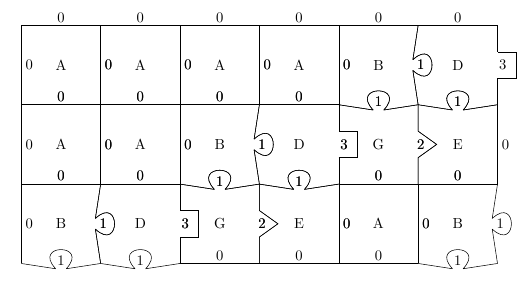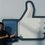# When Math meets CS: Radix Conversion = Jigsaw Puzzles!Here's a neat little (inefficient) trick: say I give you 8 types of puzzle piecespuzzle pieces

then I can arbitrarily convert any number from base 4 into base 2! Don't believe me? Here's how: say you want to convert $(301)_4 = 49_{10} = 110001_2$, what we should do is create an empty frame whose right side matches the number we need to transform in base 4:basic frame

and try to fill this frame out. It turns out that there's a unique way to fill this puzzle out, but after we're done, we can just read the type of the curve on the bottom of the puzzle to extract the base two representation:puzzle solution

notice that $110001_2 = 301_4 = 49$! What sorcery is this?

In order to understand how this works, let's look at two operators. Let $\begin{array}{c}a\\ \triangledown\\b\end{array}$ be the representation of $(ab)_4$, so for example $\begin{array}{c}2\\\triangledown\\3\\\triangledown \\ 0 \end{array} = (230)_4 = 44$ and let $a \triangleright b$ be the binary representation $(ab)_2$, so $1 \triangleright 0 \triangleright 0 \triangleright 1 = (1001)_2 = 9$ It can be easily shown that $a\triangledown b = 4a + b$ and $c \triangleright d = 2c + d$.

Hmmm, now things become a bit clearer. Consider an edge of a puzzle piece, say piece $G$:piece G $\triangleq$ $\boxed{\begin{array}{cc} \triangleright & 1 \\ &\triangledown \\ &2 \end{array}} = \boxed{\begin{array}{cc} \\ \triangledown \\ 3 & \triangleright~ 0 \end{array}}$

the horizontal edges are in reality functions that takes in the input $x$, and produces $x \triangleright n$ for whatever $n$ that edge is displaying. So the top edge of piece G denotes the function $x \mapsto x \triangleright 1 = 2x+1$ and the bottom edge denotes the function $x \mapsto x \triangleright 0 = 2x$. The vertical edges are symmetric w.r.t to $\triangledown$

It turns out that each piece denotes functional equality between a $x \triangleright \cdot \triangledown \cdot$ and a $x \triangledown \cdot \triangleright \cdot$. For example, the G piece says that $x \triangleright 1 \triangledown 2 = 4(2x+1)+2 = 2(4x+3) + 0 = x \triangledown 3 \triangleright 0$.

Therefore, our original system is just $\begin{array}{cc} \triangleright 0 \triangleright 0 \triangleright 0 \triangleright 0 \triangleright 0 \triangleright & 0 \\ &\triangledown \\ &3 \\ &\triangledown \\ &0 \\ & \triangledown \\ &1 \end{array}$ but by placing down the D piece, we're basically claiming that $\begin{array}{cc} \triangleright 0 \triangleright 0 \triangleright 0 \triangleright 0 \triangleright 0 \triangleright & 0 \\ &\triangledown \\ &3 \\ &\triangledown \\ &0 \\ & \triangledown \\ &1 \end{array} ~~~=~~~ \begin{array}{cccc} \triangleright 0 \triangleright 0 \triangleright 0 \triangleright 0 \triangleright &0 \\ &\triangledown\\ & 1 &\triangleright &1 \\ &&&\triangledown \\ &&&0 \\ &&& \triangledown \\ &&&1 \end{array}$ because the D piece says that $\triangleright 0 \triangledown 3 = \triangledown 1 \triangleright 1$. Because of this equality, we can also see that when we look at the final solution, no matter what path we take, the composition of all of the edges will all be the same function! With a little bit more analysis, we can derive nice properties about this system: each of the jigsaw piece is uniquely determined by the top and right edges and each puzzle has a unique solution, and it is the same solution such that all of the downward arrows are zeros, because the right-ward arrows composes only base 2 numbers. Therefore, our system basically reduces to a rewrite system on the language of $(\triangleright n,\triangledown m)$ and we seek a final form (a normal form if you will) where the downward arrows are all zeros.

Finally, we can now compute this via dynamic programming: notice that we only need to have the top and the right edge curves in order to uniquely determine the next piece. This means that we can progressively reduce our system into the normal form by zig-zagging:dynamic programmingNote by Lee Gao
7 years, 3 months ago

This discussion board is a place to discuss our Daily Challenges and the math and science related to those challenges. Explanations are more than just a solution — they should explain the steps and thinking strategies that you used to obtain the solution. Comments should further the discussion of math and science.

When posting on Brilliant:

• Use the emojis to react to an explanation, whether you're congratulating a job well done , or just really confused .
• Ask specific questions about the challenge or the steps in somebody's explanation. Well-posed questions can add a lot to the discussion, but posting "I don't understand!" doesn't help anyone.
• Try to contribute something new to the discussion, whether it is an extension, generalization or other idea related to the challenge.
• Stay on topic — we're all here to learn more about math and science, not to hear about your favorite get-rich-quick scheme or current world events.

MarkdownAppears as
*italics* or _italics_ italics
**bold** or __bold__ bold
- bulleted- list
• bulleted
• list
1. numbered2. list
1. numbered
2. list
Note: you must add a full line of space before and after lists for them to show up correctly
paragraph 1paragraph 2

paragraph 1

paragraph 2

[example link](https://brilliant.org)example link
> This is a quote
This is a quote
    # I indented these lines
# 4 spaces, and now they show
# up as a code block.

print "hello world"
# I indented these lines
# 4 spaces, and now they show
# up as a code block.

print "hello world"
MathAppears as
Remember to wrap math in $$ ... $$ or $ ... $ to ensure proper formatting.
2 \times 3 $2 \times 3$
2^{34} $2^{34}$
a_{i-1} $a_{i-1}$
\frac{2}{3} $\frac{2}{3}$
\sqrt{2} $\sqrt{2}$
\sum_{i=1}^3 $\sum_{i=1}^3$
\sin \theta $\sin \theta$
\boxed{123} $\boxed{123}$

## Comments

Sort by:

Top Newest

Wow.

I have no words on how awesome this is. It's just mind blown.

- 7 years, 3 months ago

Log in to reply

Haha, thanks :) I had a fun time writing this up too

- 7 years, 3 months ago

Log in to reply

after reading this, i am left with nothing except to say waaoooow! that was really amazing... at first, it was a bit unclear... but the things then came out smoothly afterwards! cheers!! :)

- 7 years, 3 months ago

Log in to reply

×

Problem Loading...

Note Loading...

Set Loading...